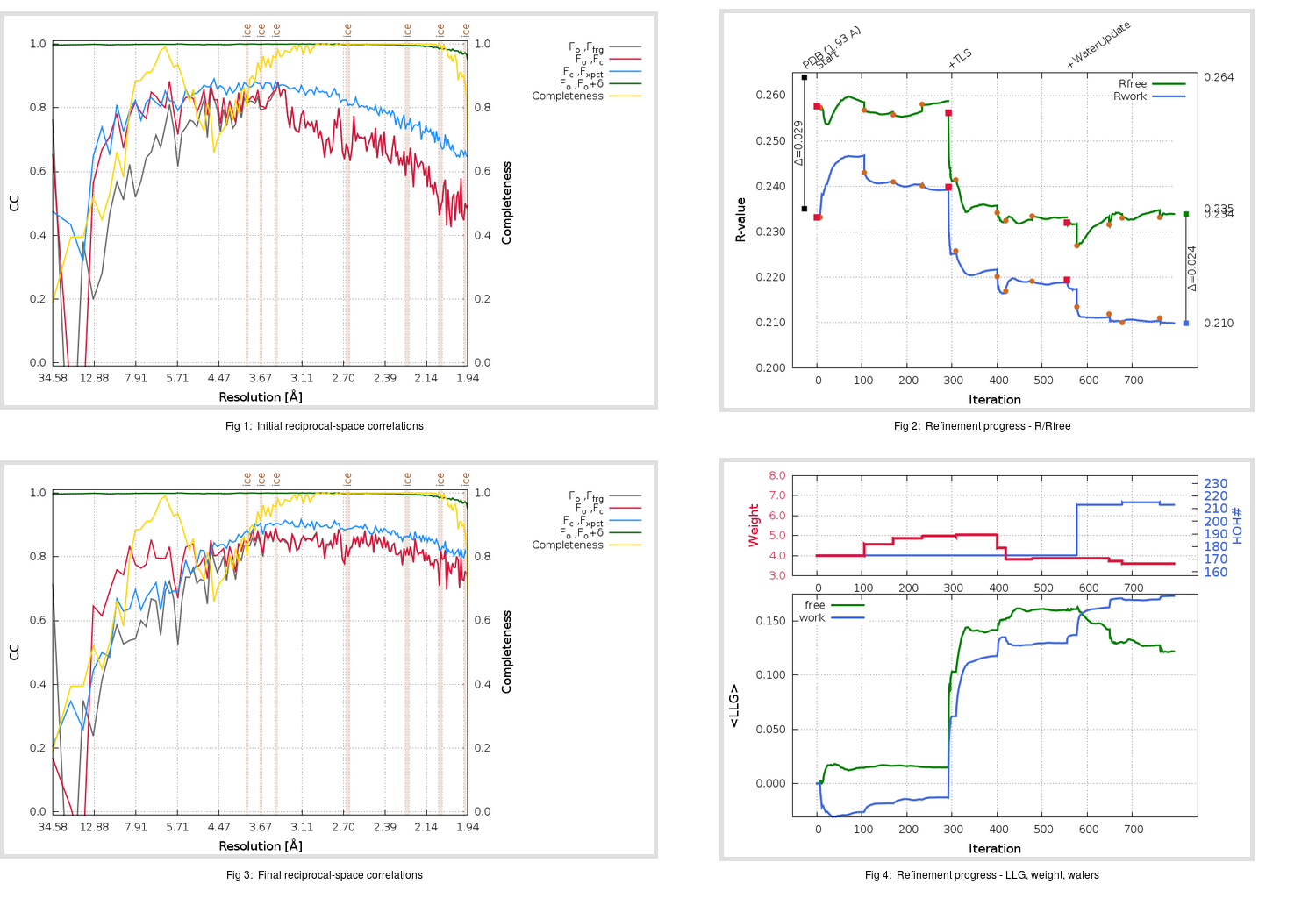Content:

```    Diffraction limits & principal axes of ellipsoid fitted to diffraction cut-off surface:
1.896         0.9503   0.0000   0.3113       0.999 a* + 0.032 c*
1.924         0.0000   1.0000   0.0000       b*
1.850        -0.3113   0.0000   0.9503      -0.537 a* + 0.844 c*
```

## Deposited

` `
 Date deposited Date data collection Resolution R, Rfree 20081012 20070614 1.93 0.2350 0.2640

Molprobity (CCP4 7.0 version) summary:

```Ramachandran outliers =   0.99 %
favored =  93.75 %
Rotamer outliers      =   6.84 %
C-beta deviations     =     1
Clashscore            =  14.63
RMS(bonds)            =   0.0184
RMS(angles)           =   2.00
MolProbity score      =   2.72
Resolution            =   1.93
R-work                =   0.2350
R-free                =   0.2640
```

```Number of waters      =   173

<B> (all atoms) =   52.32 ( sd =   13.19 ) for       2588 non-hydrogen atoms
<B>   (protein) =   51.81 ( sd =   13.22 ) for       2371 non-hydrogen atoms
<B>     (water) =   59.80 ( sd =   11.30 ) for        173 non-hydrogen atoms
<B>    (others) =   50.65 ( sd =    9.21 ) for         44 non-hydrogen atoms

B min/max       (all non-hydrogen atoms) =   25.67 /   96.39
B min/max   (protein non-hydrogen atoms) =   25.67 /   96.39
B min/max     (water non-hydrogen atoms) =   33.99 /   91.65
B min/max     (other non-hydrogen atoms) =   35.50 /   65.50
```

## BUSTER (re-)refinement

` `

Molprobity (CCP4 7.0 version) summary:

```Ramachandran outliers =   0.00 %
favored =  97.37 %
Rotamer outliers      =   5.32 %
C-beta deviations     =     1
Clashscore            =   2.72
RMS(bonds)            =   0.0111
RMS(angles)           =   1.56
MolProbity score      =   1.73
Resolution            =   1.93
R-work                =   0.2099
R-free                =   0.2339
```

```Number of waters      =   213

<B> (all atoms) =   54.92 ( sd =   14.19 ) for       2628 non-hydrogen atoms
<B>   (protein) =   54.06 ( sd =   13.63 ) for       2371 non-hydrogen atoms
<B>     (water) =   65.61 ( sd =   16.81 ) for        213 non-hydrogen atoms
<B>    (others) =   49.54 ( sd =    7.71 ) for         44 non-hydrogen atoms

B min/max       (all non-hydrogen atoms) =   31.40 /  179.18
B min/max   (protein non-hydrogen atoms) =   31.40 /  103.12
B min/max     (water non-hydrogen atoms) =   34.30 /  179.18
B min/max     (other non-hydrogen atoms) =   38.96 /   60.17
```

Refinement progression:Results:

` `
 File Remark 2ZU4_aB_refine.01_03_refine.pdb.gz exact refinement commands are in header 2ZU4_aB_refine.01_03_refine.mtz.gz including original deposited data and several re-refinement map coefficients 2ZU4_aB_refine.01_03_BUSTER_model.cif.gz including any non-standard compound restraints 2ZU4_aB_refine.01_03_BUSTER_refln.cif.gz